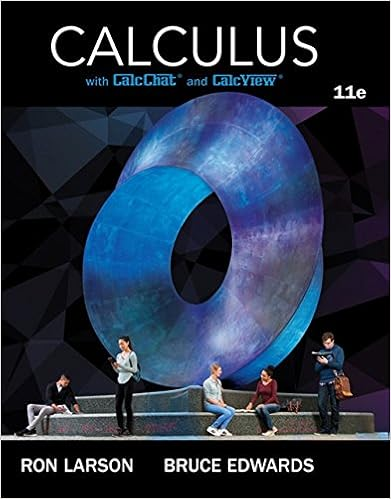# A fold a 3 inch by 5 inch index card on one of its

• 62

This preview shows page 12 - 15 out of 62 pages.

##### We have textbook solutions for you!
The document you are viewing contains questions related to this textbook.The document you are viewing contains questions related to this textbook.
Chapter 11 / Exercise 2
Calculus
Edwards/LarsonExpert Verified
a. Fold a 3-inch by 5-inch index card on one of its diagonals. 3 in. MAKING SENSE OF PROBLEMS To be proficient in math, you need to explain to yourself the meaning of a problem and look for entry points to its solution. ps 1 2 3 4 5 6 7 8 9 1 0 1 1 1 2 1 3 1 4 1 5 1 6 1 7 1 8 1 9 2 0 2 0 2 1 2 2 2 3 2 4 2 5 2 6 2 7 2 8 2 9 INCH fold 3 0 CM 1 2 3 4 5 6 7 8 9 10 11 12
##### We have textbook solutions for you!
The document you are viewing contains questions related to this textbook.The document you are viewing contains questions related to this textbook.
Chapter 11 / Exercise 2
Calculus
Edwards/LarsonExpert Verified
12 Chapter 1 Basics of Geometry What You Will Learn What You Will Learn Use the Ruler Postulate. Copy segments and compare segments for congruence. Use the Segment Addition Postulate. Using the Ruler Postulate In geometry, a rule that is accepted without proof is called a postulate or an axiom . A rule that can be proved is called a theorem , as you will see later. Postulate 1.1 shows how to find the distance between two points on a line. 1.2 Lesson Using the Ruler Postulate Measure the length of ST to the nearest tenth of a centimeter. S T SOLUTION Align one mark of a metric ruler with S . Then estimate the coordinate of T . For example, when you align S with 2, T appears to align with 5.4. S T cm 1 2 3 4 5 6 ST = 5.4 2 = 3.4 Ruler Postulate So, the length of ST is about 3.4 centimeters. Monitoring Progress Monitoring Progress Help in English and Spanish at BigIdeasMath.com Use a ruler to measure the length of the segment to the nearest 1 8 inch. 1. M N 2. P Q 3. U V 4. W X postulate, p. 12 axiom, p. 12 coordinate, p. 12 distance, p. 12 construction, p. 13 congruent segments, p. 13 between, p. 14 Core Vocabulary Core Vocabu l a ry Postulate Postulate Postulate 1.1 Ruler Postulate The points on a line can be matched one to one with the real numbers. The real number that corresponds to a point is the coordinate of the point. The distance between points A and B , written as AB , is the absolute value of the difference of the coordinates of A and B . A AB AB = x 2 x 1 B x 1 x 2 A B x 1 x 2 names of points coordinates of points
Section 1.2 Measuring and Constructing Segments 13 Constructing and Comparing Congruent Segments A construction is a geometric drawing that uses a limited set of tools, usually a compass and straightedge .
•••